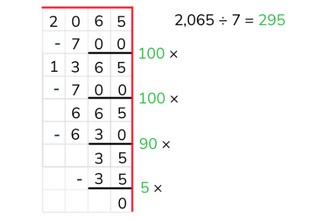Partial quotients division with a number greater than 1,000 by a number <10

# Partial quotients division with a number greater than 1,000 by a number <10

Students learn partial quotients division with a number greater than 1,000.

No account needed.8,000 schools use Gynzy92,000 teachers use Gynzy1,600,000 students use Gynzy

## General

The students learn partial quotients division with a number greater than 1,000 by a number less than 10.

## Standards

CCSS.Math.Content.4.NBT.B.6

## Learning objective

Students will be able to use partial quotients to divide a number greater than 1,000 by a number less than 10.

## Introduction

On the interactive whiteboard there are a few problems, for which a child states what the answer is. The students must solve each problem using partial quotients to determine whether the statement of each child is correct or not.

## Instruction

Explain that with partial quotients division you break the problem up into pieces and then solve it in this way. You write down the problem and draw a line underneath. Next you can use a helping row from the table of the number that you are dividing by. You don't have to write down every problem in this row. You can double or halve certain problems, or imagine a 0 at the end of them. After that you solve the problem step by step to see how many times 5 fits in 1,120. After every step you write down how much you have left over. If you don't have anything else left, then the problem is finished. You add all of the numbers that give how many times 5 fits in 1,120 (100, 100, 20, and 4) together. Have the students solve a number of problems using partial quotients. Have them write down the problems on graphing paper, and include a helping row if they need it. Encourage the students to take as large steps as they can.

Check whether the students can use partial quotients to divide by asking the following questions:
- Why is it useful to be able to divide using partial quotients?
- What aid can you use when dividing using partial quotients?
- Solve this problem using partial quotients: 7,410 ÷ 6

## Quiz

The students test their understanding of partial quotients division with a number greater than 1,000 by a number less than 10, through ten exercises. Each of the exercises is a division problem. The students should write down the problems on graphing paper to solve using partial quotients.They can also write down the table of the divisor if they need to.

## Closing

You discuss with the students once again how it is important to be able to divide using partial quotients with a number greater than 1,000 by a number less than 10, because that is how you can solve a problem in a simpler way. Check if the students know that with partial quotients division you solve pieces of the problem in steps, and that they can use a helping row to accomplish this. On the interactive whiteboard there is a short story. Ask the students what problem matches the story and then have them solve the problem using partial quotients division.

## Teaching tips

When students have difficulty with partial quotients division, you can have them make helping rows from the table of the number that you are dividing by. Have them solve each problem step by step, until there is nothing left. Check each of the steps they take and encourage them to take larger steps (not 100 × 3 = 300 and 100 × 3 = 300, but in one step 200 × 3 = 600).

## Instruction materials

Graphing paper

### The online teaching platform for interactive whiteboards and displays in schools

• Save time building lessons

• Manage the classroom more efficiently

• Increase student engagement